## Integration by Parts

A first-order (single) integration by parts uses(1)(2)

so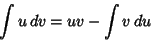(3)

and(4)

Now apply this proceduretimes to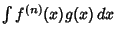.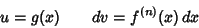(5)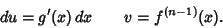(6)

Therefore,(7)

But(8)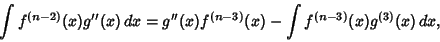(9)

so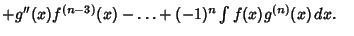(10)
Now consider this in the slightly different form. Integrate by parts a first time(11)(12)

so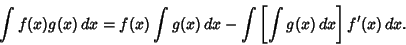(13)

Now integrate by parts a second time,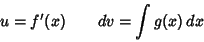(14)(15)

so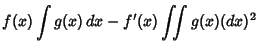(16)

Repeating a third time,(17)

Therefore, afterapplications,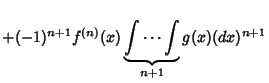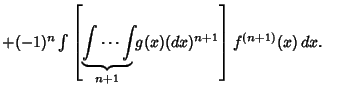(18)
If(e.g., for anth degree Polynomial), the last term is 0, so the sum terminates afterterms and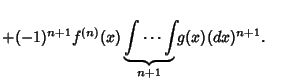(19)

References

Abramowitz, M. and Stegun, C. A. (Eds.). Handbook of Mathematical Functions with Formulas, Graphs, and Mathematical Tables, 9th printing. New York: Dover, p. 12, 1972.